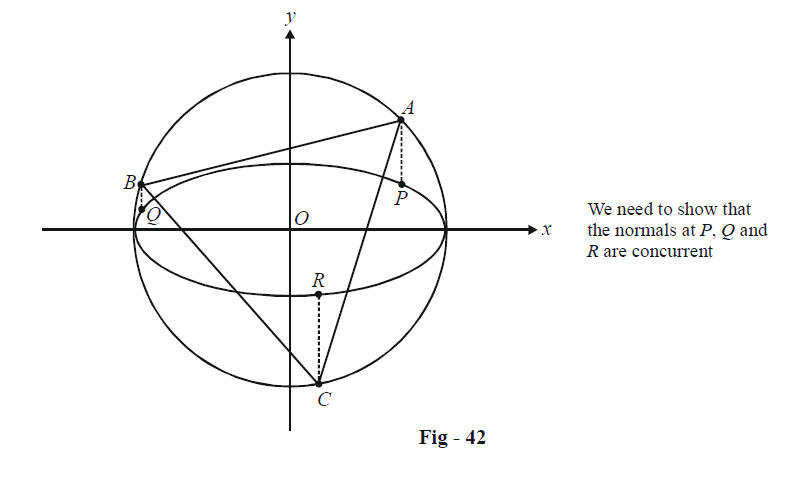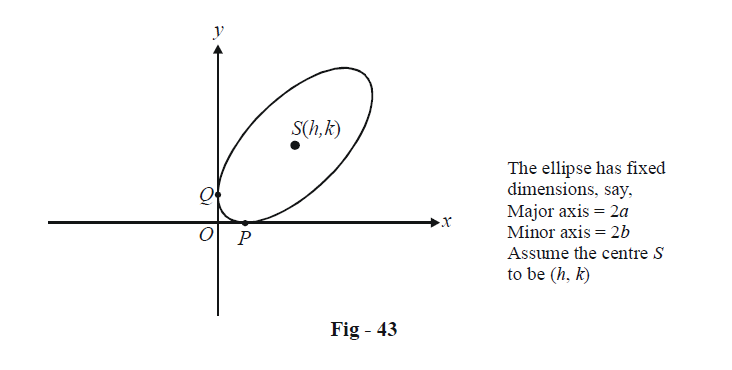# Ellipses Set-3

Go back to  'SOLVED EXAMPLES'

Example - 5

Let ABC be an equilateral triangle inscribed in the circle $${x^2} + {y^2} = {a^2}.$$ Perpendiculars from A, B, C to the major axis of the ellipse $$\frac{{{x^2}}}{{{a^2}}} + \frac{{{y^2}}}{{{b^2}}} = 1$$ (where a > b) meet the ellipse respectively at P, Q, R so that P, Q and R lie on the same side of the major axis of A, B and C respectively.Prove that the normals to the ellipse at P, Q and R are concurrent.

Solution: The following figure graphically portrays the situation described in the question:All the information given about ABC being equilateral and all can be reduced to this single piece of significant information: the polar angles of A, B and C, and hence, the eccentric angles of P, Q and R, will be evenly spaced at $$\frac{{2\pi }}{3},$$ by virtue of $$\Delta ABC$$ being equilateral.

Thus, we can assume the eccentric angels of P, Q and R to be $$\theta ,\,\,\theta + \frac{{2\pi }}{3},\,\,\theta - \frac{{2\pi }}{3}.$$

Now, the equation of a normal to $$\frac{{{x^2}}}{{{a^2}}} + \frac{{{y^2}}}{{{b^2}}} = 1$$ at eccentric angle $$\phi$$ is given by

\begin{align}&\qquad ax\sec \phi - by\cos ec\phi = {a^2} - {b^2}.\\& \Rightarrow \quad~~ ax\sin \phi - by\cos \phi = \frac{{({a^2} - {b^2})\sin 2\phi }}{2}.\end{align}

Thus, the normals at P, Q and R are respectively given by

\begin{align}&{N_P}\quad : \quad ax\sin \theta - by\cos \theta = \frac{{({a^2} - {b^2})\sin 2\theta }}{2}\\&{N_Q}\quad :\quad ax\sin \left( {\theta + \frac{{2\pi }}{3}} \right) - by\cos \left( {\theta + \frac{{2\pi }}{3}} \right) = \frac{{\left( {{a^2} - {b^2}} \right)}}{2}\sin \left( {2\theta + \frac{{4\pi }}{3}} \right)\\&{N_R} \quad : \quad ax\sin \left( {\theta - \frac{{2\pi }}{3}} \right) - by\cos \left( {\theta - \frac{{2\pi }}{3}} \right) = \frac{{({a^2} - {b^2})}}{2}\sin \left( {2\theta - \frac{{4\pi }}{3}} \right)\end{align}

Let us evaluate $$\Delta ,$$ the determinant of the coefficients of these three equations:

\Delta =\frac{ab({{a}^{2}}-{{b}^{2}})}{2}\left| \begin{align}&\qquad \sin \theta &&\qquad \cos \theta &&\qquad \sin 2\theta \\& \sin \left( \theta +\frac{2\pi }{3} \right) && \cos \left( \theta +\frac{2\pi }{3} \right) && \sin \left( 2\theta +\frac{4\pi }{3} \right) \\& \sin \left( \theta -\frac{2\pi }{3} \right) && \cos \left( \theta -\frac{2\pi }{3} \right) & &\sin \left( 2\theta -\frac{4\pi }{3} \right) \\\end{align} \right|

Using the row operation $${R_1} \to {R_1} + {R_2} + {R_3},$$ the first row reduces to zero, which means that

$\Delta = 0$

Thus, the normals at P, Q and R must be concurrent.

Example – 6

An ellipse slides between two lines at right angles to one another. Show that the locus of its centre is a circle.

Solution: It would be easiest to assume the two lines to be the coordinate axis, and an ellipse of fixed dimensions sliding between these two lines as shown below:From the view-point of the ellipse, since the tangents to it at P and Q intersect at right angles at O, the point O must lie on the director circle of the ellipse. Since the radius of the director circle is $$\sqrt {{a^2} + {b^2}} ,$$ we must have

\begin{align}&\qquad\quad OS = \sqrt {{a^2} + {b^2}} \\ &\Rightarrow \quad O{S^2} = {a^2} + {b^2}\\& \Rightarrow \quad {h^2} + {k^2} = {a^2} + {b^2}\end{align}

This must be the locus of the centre S! It can be written in x – y form as

${x^2} + {y^2} = {a^2} + {b^2}$

It is evident that this is a circle centred at the origin and of radius $$\sqrt {{a^2} + {b^2}} .$$

Learn from the best math teachers and top your exams

• Live one on one classroom and doubt clearing
• Practice worksheets in and after class for conceptual clarity
• Personalized curriculum to keep up with school